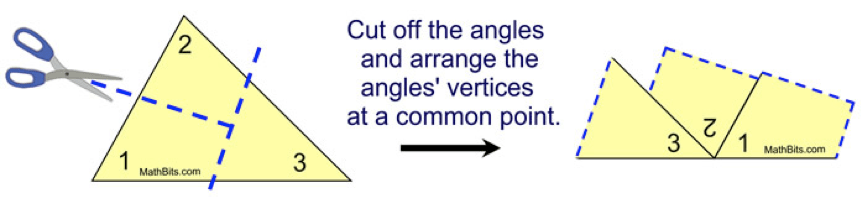# GEOM 2 | Lesson 1 | Try This! (Sums of Triangle Interior Angles)## Sums of Triangle Interior Angles

Follow the steps below to see one example to show how the sum of the three interior angles of a triangle is determined.

1. Find a printed copy of a scalene triangle, or draw one on a sheet of paper. Label each angle as 1, 2, and 3.
2. Cut the triangle out, and tear or cut each of the three corners (see figure below).
3. Draw a horizontal line, and arrange the triangle corners so that they sit on this line as shown below.• What is the angle measure of a line which is also called a straight angle?

• If all three angles from the triangle form a line, then what is the sum of the angles in a triangle?